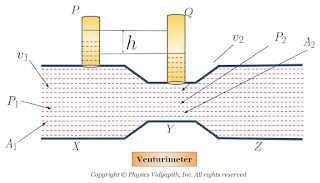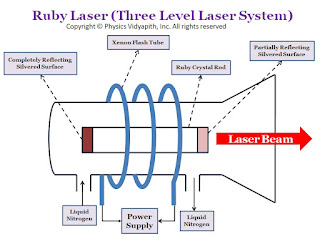Showing posts with label Properties of Matter. Show all posts
Showing posts with label Properties of Matter. Show all posts

## Principle, Construction and Working of Venturimeter

Principle of Venturimeter:

It is a device that is used for measuring the rate of flow of liquid through pipes. Its principle and working are based on Bernoulli's theorem and equation.

Construction:

It consists of two identical coaxial tubes $X$ and $Z$ connected by a narrow co-axial tube $Y$. Two vertical tubes $P$ and $Q$ are mounted on tubes $X$ and $Y$ to measure the pressure of the liquid that flows through pipes.. As shown in the figure below.Working and Theory:

Connect this venturimeter horizontally to the pipe through which the liquid is flowing and note down the difference of liquid columns in tubes $P$ and $E$. Let the difference is $h$.

Let us consider that an incompressible and non-viscous liquid flows in streamlined motion through a tube $X$,$Y$, and $Z$ of the non-uniform cross-section.

Now Consider:

The Area of cross-section of tube $X$ = $A_{1}$
The Area of cross-section of tube $Y$ = $A_{2}$

The velocity per second (i.e. equal to distance) of fluid at the cross-section of tube $X$ = $v_{1}$
The velocity per second (i.e. equal to distance) of fluid at the cross-section of tube $Y$ = $v_{2}$

The Pressure of fluid at cross-section of tube $X$ = $P_{1}$
The Pressure of fluid at cross-section of tube $Y$ = $P_{2}$

Now the change in pressure on tube $P$ and $Q$:

$P_{1}-P_{2}=\rho g h \qquad(1)$

Where $\rho$ is the density of liquid.

According to the principle of continuity:

$A_{1} v_{1} = A_{2} v_{2} = \frac{m}{\rho}= V \qquad(2)$

Where $V$ is the volume of the liquid flowing per second through the pipe. Then from equation $(2)$

$\left.\begin{matrix} v_{1}=\frac{V}{A_{1}} \\ v_{2}=\frac{V}{A_{2}} \end{matrix}\right\} \qquad(3)$

Now apply Bernoulli's Theorem for horizontal flow (i.e $h_{1}=h_{2}$) in verturimeter.

$P_{1} + \frac{1}{2} \rho v_{1}^{2} = P_{2} + \frac{1}{2}\rho v_{2}^{2}$

$P_{1} - P_{2} = \frac{1}{2}\rho v_{2}^{2} - \frac{1}{2} \rho v_{1}^{2}$

$P_{1} - P_{2} = \frac{1}{2}\rho \left( v_{2}^{2} - v_{1}^{2} \right) \qquad(4)$

Now from equation $(1)$ and equation $(4)$, we get

$\rho g h = \frac{1}{2}\rho \left( v_{2}^{2} - v_{1}^{2} \right)$

$g h = \frac{1}{2} \left( v_{2}^{2} - v_{1}^{2} \right)$

Now substitute the value of $v_{1}$ and $v_{2}$ from equation $(3)$ in above equation

$g h = \frac{1}{2} \left( \frac{V^{2}}{A^{2}_{2}} - \frac{V^{2}}{A^{2}_{1}} \right)$

$g h = \frac{V^{2}}{2} \left( \frac{1}{A^{2}_{2}} - \frac{1}{A^{2}_{1}} \right)$

$g h = \frac{V^{2}}{2} \left( \frac{ A^{2}_{1} - A^{2}_{2} }{A^{2}_{1} A^{2}_{2}} \right)$

$V^{2} = \frac{2 g h A^{2}_{1} A^{2}_{2}}{A^{2}_{1} - A^{2}_{2}}$

$V = A_{1} A_{2} \sqrt{\frac{2 g h }{A^{2}_{1} - A^{2}_{2}}}$

Hence flow rate of liquid can be calculated by measuring $h$, since $A_{1}$ and $A_{2}$ are known for the given venturimeter.

## Bernoulli's Theorem and Derivation of Bernoulli's Equation

Statement of Bernoulli's Theorem:
When an ideal fluid (i.e incompressible and non-viscous Liquid or Gas) flows in streamlined motion from one place to another, then the total energy per unit volume (i.e Pressure energy + Kinetic Energy + Potential Energy) at each and every of its path is constant.

$P+\frac{1}{2}\rho v^{2} + \rho gh= constant$

Derivation of Bernoulli's Theorem Equation:

Let us consider that an incompressible and non-viscous liquid is flowing in streamlined motion through a tube $XY$ of the non-uniform cross-section. Now Consider:

The Area of cross-section $X$ = $A_{1}$
The Area of cross-section $Y$ = $A_{2}$

The velocity per second (i.e. equal to distance) of fluid at cross-section $X$ = $v_{1}$
The velocity per second (i.e. equal to distance) of fluid at cross-section $Y$ = $v_{2}$

The Pressure of fluid at cross-section $X$ = $P_{1}$
The Pressure of fluid at cross-section $Y$ = $P_{2}$

The height of cross-section $X$ from surface = $h_{1}$
The height of cross-section $Y$ from surface = $h_{2}$

The work done per second by force on the liquid Entering the tube at $X$:

$W_{1}$ = Force $\times$ Distance covered in one second
$W_{1}= P_{1} \times A_{1} \times v_{1} \quad \left( Force =Pressure \times Area \right)$

Similarly
The work done per second by force on the liquid leaving the tube at $Y$:

$W_{2}= P_{2} \times A_{2} \times v_{2}$

The net work done on the liquid:

$\Delta W=W_{1}-W_{2}$
$\Delta W= P_{1} \times A_{1} \times v_{1} - P_{2} \times A_{2} \times v_{2} \qquad(1)$

Now according to the principle of continuity:

$A_{1} v_{1} = A_{2} v_{2} = \frac{m}{\rho} \qquad(2)$

Now from equation $(1)$ and equation $(2)$

$\Delta W=\left( P_{1} -P_{2} \right) \frac {m}{\rho} \qquad(3)$

The kinetic energy of the fluid entering at $X$ in 1 second

$K_{1}=\frac{1}{2}mv_{1}^{2}$

The kinetic energy of the fluid leaving at $Y$ in 1 second

$K_{2}=\frac{1}{2}mv_{2}^{2}$

Therefore, The increase in kinetic energy

$\Delta K = K_{2}-K_{1}$

Now substitute the value of $K_{1}$ and $K_{2}$ in above equation then

$\Delta K = \frac{1}{2}mv_{2}^{2} - \frac{1}{2}mv_{1}^{2}$
$\Delta K = \frac{1}{2}m \left( v_{2}^{2} - v_{1}^{2} \right) \qquad(4)$

The potential energy of fluid at $X$

$U_{1}= mgh_{1}$

The potential energy of fluid at $Y$

$U_{2}= mgh_{2}$

Therefore, The decrease in potential energy

$\Delta U = U_{1}-U_{2}$

Now substitute the value of $U_{1}$ and $U_{2}$ in above equation then

$\Delta U = mgh_{1} - mgh_{2}$
$\Delta U = mg \left( h_{1} - h_{2} \right) \qquad(5)$

This increase in energy is due to the net work done on the fluid, i.e.

Net Work done = Net increase in energy

Net Work done $(\Delta W)$ = Net increase in Kinetic Energy $(\Delta K)$ - Net decrease in Potential Energy $(\Delta U)$

$\left( P_{1} -P_{2} \right) \frac {m}{\rho} = \frac{1}{2}m \left( v_{2}^{2} - v_{1}^{2} \right) - mg \left( h_{1} - h_{2} \right)$

$\left( P_{1} -P_{2} \right) = \frac{1}{2}\rho \left( v_{2}^{2} - v_{1}^{2} \right) -\rho g \left( h_{1} - h_{2} \right)$

$P_{1} + \frac{1}{2} \rho v_{1}^{2} + \rho g h_{1} = P_{2} + \frac{1}{2}\rho v_{2}^{2} + \rho g h_{2}$

$P + \frac{1}{2} \rho v^{2} + \rho g h = Constant$

This is Bernoulli's theorem equation.

According to Bernoulli's Theorem equation

$P + \frac{1}{2} \rho v^{2} + \rho g h = Constant$

Now dividing the above equation by $\rho g$, then we get

$\frac{P}{\rho g}+\frac{v^{2}}{2g}+ h = Constant$

Where

$\frac{P}{\rho g}$ = Pressure Head

$\frac{v^{2}}{2g}$ = Velocity Head

$h$ = Gravitational Head

The dimension of each of these three is the dimension of height. The sum of these heads is called the 'Total Head'

Therefore, Bernoulli's theorem may also state as follows:

In streamlined motion of an ideal fluid, the sum of pressure head, velocity head and gravitational head at any point is always constant.

When the fluid flows in a horizontal plane $(h_{1}=h_{2})$, then Bernoulli's equation

$P_{1} + \frac{1}{2} \rho v_{1}^{2} = P_{2} + \frac{1}{2}\rho v_{2}^{2}$

$\frac{P}{\rho g}+\frac{v^{2}}{2g} = Constant$

### Principle, Construction and Working of the Ruby Laser